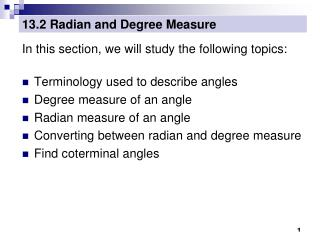Download Presentation# 13.2 Radian and Degree Measure - PowerPoint PPT PresentationDownload Presentation## 13.2 Radian and Degree Measure

- - - - - - - - - - - - - - - - - - - - - - - - - - - E N D - - - - - - - - - - - - - - - - - - - - - - - - - - -
##### Presentation Transcript

1. 13.2 Radian and Degree Measure In this section, we will study the following topics: Terminology used to describe angles Degree measure of an angle Radian measure of an angle Converting between radian and degree measure Find coterminal angles

2. 13.2 Radian and Degree Measure Angles Trigonometry: measurement of triangles Angle Measure

3. 13.2 Radian and Degree Measure Standard Position Vertex at origin The initial side of an angle in standard position is always located on the positivex-axis.

4. 13.2 Radian and Degree Measure Positive and negative angles When sketching angles, always use an arrow to show direction.

5. 13.2 Radian and Degree Measure Measuring Angles The measure of an angle is determined by the amount of rotation from the initial side to the terminal side. There are two common ways to measure angles, in degrees and in radians. We’ll start with degrees, denoted by the symbol º. One degree (1º) is equivalent to a rotation of of one revolution.

6. 13.2 Radian and Degree Measure Measuring Angles

7. 13.2 Radian and Degree Measure Classifying Angles Angles are often classified according to the quadrant in which their terminal sides lie. Ex1: Name the quadrant in which each angle lies. 50º 208º II I -75º III IV Quadrant 1 Quadrant 3 Quadrant 4

8. 13.2 Radian and Degree Measure Classifying Angles Standard position angles that have their terminal side on one of the axes are called quadrantal angles. For example, 0º, 90º, 180º, 270º, 360º, … are quadrantal angles.

9. 13.2 Radian and Degree Measure Coterminal Angles Angles that have the same initial and terminal sides are coterminal. Angles  and  are coterminal.

10. 13.2 Radian and Degree Measure Example of Finding Coterminal Angles You can find an angle that is coterminal to a given angle  by adding or subtracting multiples of 360º. Ex 2: Find one positive and one negative angle that are coterminal to 112º. For a positive coterminal angle, add 360º : 112º + 360º = 472º For a negative coterminal angle, subtract 360º: 112º - 360º = -248º

11. Ex 3. Find one positive and one negative angle that is coterminal with the angle  = 30° in standard position. Ex 4. Find one positive and one negative angle that is coterminal with the angle  = 272 in standard position.

12. 13.2 Radian and Degree Measure Radian Measure A second way to measure angles is in radians. Definition of Radian: One radian is the measure of a central angle  that intercepts arc s equal in length to the radius r of the circle. In general,

15. 13.2 Radian and Degree Measure Conversions Between Degrees and Radians • To convert degrees to radians, multiply degrees by • To convert radians to degrees, multiply radians by

16. Ex 5. Convert the degrees to radian measure. 60 30 -54 -118 45

17. Ex 6. Convert the radians to degrees. a) b) c) d)

18. Ex 7. Find one positive and one negative angle that is coterminal with the angle  = in standard position. Ex 8. Find one positive and one negative angle that is coterminal with the angle  = in standard position.

19. Degree and Radian Form of “Special” Angles 90 °   120 ° 60 °   135 ° 45 °   150 ° 30 °   0°  360 °   180 °  210 ° 330 °   225 ° 315 °   240 ° 300 °  270 ° 